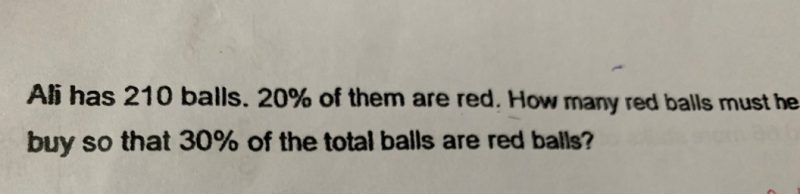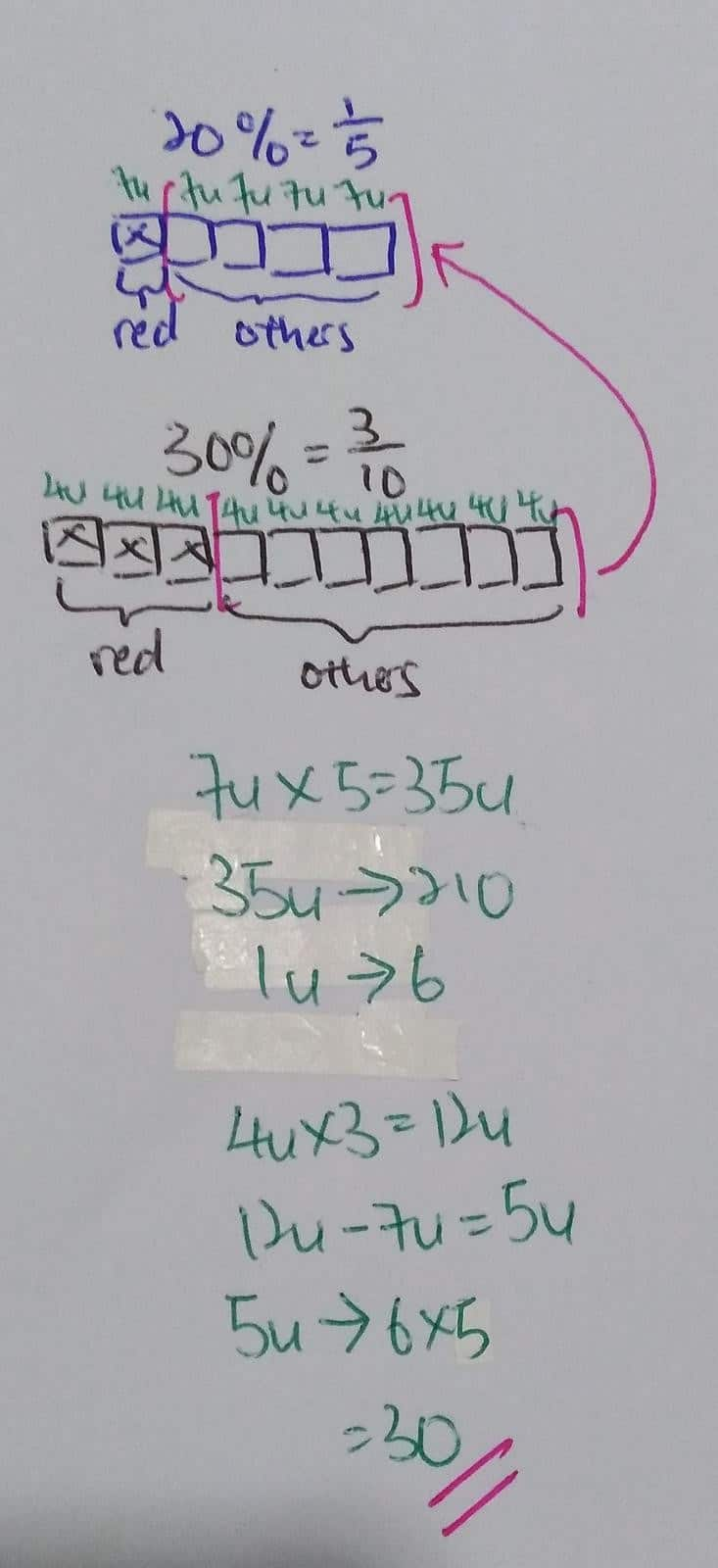# Questionuse simple way to do it, key to this question is the number of other colors of the balls is UNCHANGED.

at beginning,  red is 42 (20%), other color is 168

after that rea is 30%, other color is 70% -> 168, so total number of balls now(100%) is 240, red ball is 72, so need to have 72 – 42 = 30 red balls added.

or simply 240 – 210 = 30 red ball needed.

0 Replies 1 Like ✔Accepted Answer